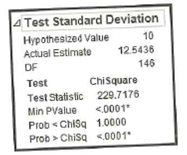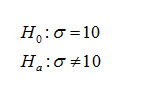# 4 Test Standard DeviationHypothesized ValueActual Estimate1012.5436146DFChiSquare229.7176 Chisq

Question
1 views

Pulse Rates of Women Repeat the preceding exercise using the pulse rates of women listed in Data Set 1 “Body Data” in Appendix B. For the sample of pulse rates of women, n = 147 and s = 12.5. See the accompanying JMP display that results from using the original list of pulse rates instead of the summary statistics. (Hint: The bottom three rows of the display provide P-values for a two-tailed test, a left-tailed test, and a right-tailed test, respectively.) What do the results indicate about the effectiveness of using the range rule of thumb with the “normal range” from 60 to 100 beats per minute for estimating σ in this case?help_outlineImage Transcriptionclose4 Test Standard Deviation Hypothesized Value Actual Estimate 10 12.5436 146 DF ChiSquare 229.7176 <.0001 Prob < Chisq 1.0000 Prob > Chisq <.0001 Test Test Statistic Min PValue fullscreen
check_circle

Step 1

The sample standard deviation and the sample size are, s = 12.5 and n = 147 respectively. The sample mean is 12.19. The null and alternative hypotheses are given below:Step 2

From the output, the test statistic value is 229.7176.  Assume that the level of significance is 0.01. The p-value of the test is less than 0.0001.

Decision rule:

If p-value ≤α, then reject the null hypothesis. Otherwise, do not reject the null hypothesis.

Conclusion:

Here, p-value (less than 0.0001) is less than the level of significance (0.0...

### Want to see the full answer?

See Solution

#### Want to see this answer and more?

Solutions are written by subject experts who are available 24/7. Questions are typically answered within 1 hour.*

See Solution
*Response times may vary by subject and question.
Tagged in